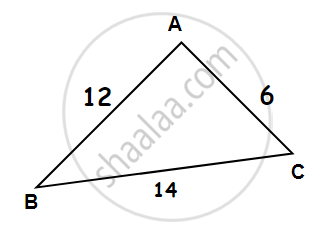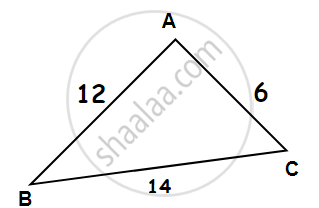# Property of the lengths of sides of a triangle:

## 1. Sum of the lengths of two sides of a triangle:

The sum of the lengths of any two sides of a triangle is greater than the length of the third side.In the above triangle,
6 + 12 = 18 > 14
12 + 14 = 26 > 6
6 + 14 = 20 > 12

## 2. Difference between lengths of two sides of a triangle:

The difference between the lengths of any two sides is smaller than the length of the third side.In the above triangle,
12 – 6 = 6 < 14
14 – 12 = 2 < 6
14 – 6 = 8 < 12.

#### Example

Is there a triangle whose sides have lengths 10.2 cm, 5.8 cm, and 4.5 cm?

Suppose such a triangle is possible.
Then the sum of the lengths of any two sides would be greater than the length of the third side.
4.5 + 5.8 >10.2
5.8 + 10.2 > 4.5
10.2 + 4.5 > 5.8
Therefore, the triangle is possible.

#### Example

The lengths of two sides of a triangle are 6 cm and 8 cm. Between which two numbers can the length of the third side fall?

We know that the sum of two sides of a triangle is always greater than the third.

Therefore, the third side has to be less than the sum of the two sides. The third side is thus,less than 8 + 6 = 14 cm.

The side cannot be less than the difference between the two sides. Thus, the third side has to be more than 8 – 6 = 2 cm.

The length of the third side could be any length greater than 2 and less than 14 cm.

If you would like to contribute notes or other learning material, please submit them using the button below.

### Shaalaa.com

Sum of the lengths of Two Sides of a Triangle [00:10:23]
S
0%